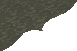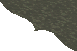Proceedings of the
Organic Mathematics
Workshop

Help: A Guide to Organics

Hypertext, such as above, and hyperimages, such as the various icons below, appear throughout the pages of the Proceedings. Hypertext Menus are used to allow quick navigation through the proceedings hierarchy. Hyperimages are used for accessing two specialized and customizable Web interfaces from any desired point within a given paper: the Maple Form Interface and the Annotation Form Interface.

This is an interactive help page which summarizes the various icons used to access the MFI and AFI. Click on any of the hypertext and icons described in this page for an illustration of the associated action.

• MathActivation Icons
• The Red Maple Icon: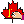As a hyperimage appearing to the right or left of typeset mathematical expressions, this icon indicates that the corresponding expression(s) is MathActivatable, i.e., there are author-specified Maple code attached to the equation. Clicking on this icon in the context of a document will results in the interpretation of the associated Maple source via the Maple Form Interface (MFI). Try clicking on the Maple icon below to activate a Maple program for graphing arbitrary functions on the torus: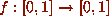• The Blue Maple Icon: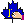This hyper image appears to the left of a typeset mathematical expression if there is no Maple code attached to the expression(s) by the author. Try clicking on the icon to see how it would typically appear in a typical document. Clicking on the blue Maple icon within the context of a document will provide access to the generic MFI.

• The Equation Icon:Click on any in-lined image of an equation, such as the arbitrary function above, to view three different representations of the equation:

• The typeset image of the equation with the Maple icon
• The corresponding LaTeX code used to typeset the equation
• The corresponding Maple code used for Mathactivation
Note that the so-called Equation Icon is the only icon which changes (since the image representing the various typeset mathematical expressions changes). In addition, a given Equation Icon (with exactly the same pixmap) in a document is context sensitive with different corresponding mathactivations (Maple code).

• Annotation Icons

• The Help IconClick on this icon to view this page and any author supplied comments/help specific to a particular section of the document.

At the bottom of almost all pages there is a navigation bar (like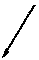the one at the bottom of this page). It provides point-and-click access to all the main sections of the Proceedings: